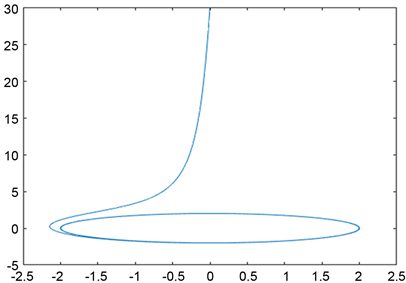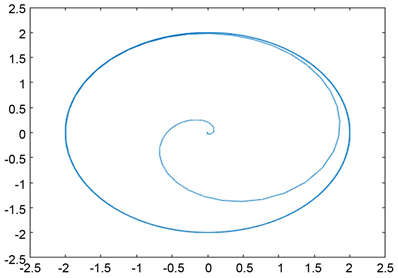﻿ 车辆动态竞争系统的动力学分析

# 车辆动态竞争系统的动力学分析Dynamic Analysis of Vehicle Dynamic Competition System

Abstract: Based on the models and methods of applying ecology theory to the study of enterprise competition, this paper improves the LV model in order to solve vehicle competition problem in the research of regional logistics transportation system. At first, taking two teams as the research objects, the vehicle dynamic competition model is established. Secondly, according to the analysis of the type of equilibrium point, the number of the two teams is concluded. As a result of the disturbance of competition system, we get a stable limited cycle, and make a reasonable explanation about the limited cycle. In this paper, numerical simulation was carried out on the model test to ensure the rationality of the model. In the end, combined with the law of social and economic development, this paper makes relevant judgment and prediction on the development of transport fleet in regional logistics.

1. 引言

2. 车辆动态竞争系统模型的构建

20世纪20年代，意大利生物学家U. D’Ancona在研究鱼类变化规律时，得到了第一次世界大战期间意大利Finme港收购站的软骨食肉鱼收购量的数据，并拿去请教了当时的同事，数学家V. Volterra。在假设种群数量的变化只依赖于两个种群的规模，不受其他种群和环境状态的影响的条件下，Volterra以此得到了捕食种群与食饵种群相互作用的数学模型，之后又得到了两种群相互作用的Lotka-Volterra模型，其一般形式为：

$\left\{\begin{array}{l}\frac{\text{d}x}{\text{d}t}=x\left({a}_{1}+{b}_{1}x+{c}_{1}y\right)\\ \frac{\text{d}y}{\text{d}t}=y\left({a}_{2}+{b}_{2}x+{c}_{2}y\right)\end{array}$(2.1)

$\left\{\begin{array}{c}\frac{\text{d}x}{\text{d}t}={r}_{1}x\left(1-\frac{x}{M}\right)-{l}_{1}xy-{k}_{1}y\\ \frac{\text{d}y}{\text{d}t}={r}_{2}y\left(1-\frac{y}{N}\right)-{l}_{2}xy-{k}_{2}x\end{array}$(2.2)

3. 车辆动态竞争系统平衡点分析

$\left\{\begin{array}{l}\frac{\text{d}x}{\text{d}t}={r}_{1}x-{k}_{1}y-\frac{{r}_{1}{x}^{2}}{M}-\frac{{r}_{1}}{M}xy\\ \frac{\text{d}y}{\text{d}t}=-{k}_{2}x+{r}_{2}y-\frac{{r}_{2}{y}^{2}}{N}-\frac{{r}_{2}xy}{N}\end{array}$(3.1)

$\left\{\begin{array}{l}{r}_{1}x-{k}_{1}y-\frac{{r}_{1}{x}^{2}}{M}-\frac{{r}_{1}xy}{M}=0\\ -{k}_{2}x+{r}_{2}y-\frac{{r}_{2}{y}^{2}}{N}-\frac{{r}_{2}xy}{N}=0\end{array}$(3.2)

3.1. 平衡点为原点的动力学分析

$\left\{\begin{array}{l}\frac{\text{d}x}{\text{d}t}={r}_{1}x-{k}_{1}y\\ \frac{\text{d}y}{\text{d}t}={r}_{2}y-{k}_{2}x\end{array}$(3.3)

${\lambda }_{1}=\frac{{r}_{1}+{r}_{2}+\sqrt{{\left({r}_{1}-{r}_{2}\right)}^{2}+4{k}_{1}{k}_{2}}}{2}$${\lambda }_{2}=\frac{{r}_{1}+{r}_{2}-\sqrt{{\left({r}_{1}-{r}_{2}\right)}^{2}+4{k}_{1}{k}_{2}}}{2}$

${\lambda }_{1}>0$ 是显然成立的，那么我们只需对 ${\lambda }_{2}$ 讨论即可。

${r}_{1}{r}_{2}>{k}_{1}{k}_{2}$ 时， ${\lambda }_{2}>0$ ，此时 ${\lambda }_{1}>{\lambda }_{2}>0$ ，平衡点 $O\left(0,0\right)$ 为不稳定结点，此时两家车队的服务数量都在增加，说明在两家车队在竞争中相互促进发展。当 ${r}_{1}{r}_{2}<{k}_{1}{k}_{2}$ 时，说明车辆运输企业在发展到一定阶段时，自身的增长率远小于竞争系数，主要通过竞争来壮大自己的实力和服务队伍。因此 ${\lambda }_{2}<0<{\lambda }_{1}$ ，平衡点变为鞍点，此时一方物流运输车队服务数量的增加，将会导致另一方服务数量的减少，呈现出一方吞并的发展趋势。

3.2. 平衡点不为原点时的动力学分析 

$X\left(x,y\right)={r}_{1}x\left(1-\frac{x}{M}\right)-{k}_{1}y-\frac{{r}_{1}}{M}xy$$Y\left(x,y\right)={r}_{2}y\left(1-\frac{y}{N}\right)-{k}_{2}x-\frac{{r}_{2}}{N}xy$ 。那么,

$\frac{\partial X}{\partial x}={r}_{1}-\frac{2{r}_{1}}{M}x-\frac{{r}_{1}}{M}y$$\frac{\partial X}{\partial y}=-{k}_{1}-\frac{{r}_{1}x}{M}$ , $\frac{\partial Y}{\partial x}=-{k}_{2}-\frac{{r}_{2}}{N}y$$\frac{\partial Y}{\partial y}={r}_{2}-\frac{2{r}_{2}y}{N}-\frac{{r}_{2}x}{N}$

1) 当平衡点为 $A\left(M,0\right)$ 时，系统(3.1)的线性近似系统的系数矩阵为

${A}_{1}=\left(\begin{array}{cc}\begin{array}{c}-{r}_{1}\\ -{k}_{2}\end{array}& \begin{array}{c}-{k}_{1}-{r}_{1}\\ {r}_{2}\left(1-M/N\right)\end{array}\end{array}\right)$

$\Delta ={\left({r}_{1}+{r}_{2}\right)}^{2}+\frac{{r}_{2}M}{N}\left(\frac{{r}_{2}M}{N}-2{r}_{1}-2{r}_{2}\right)+4{k}_{2}\left({r}_{1}+{k}_{1}\right)$ 。下面对平衡点的类型进行分析：

① 当 $\Delta >0$ 时，此时有两个互异的特征根 ${\mu }_{1}$${\mu }_{2}$ ，即

${\mu }_{1}=\frac{\left(-{r}_{2}M/N+{r}_{2}-{r}_{1}\right)+\sqrt{\Delta }}{2}$${\mu }_{2}=\frac{\left(-{r}_{2}M/N+{r}_{2}-{r}_{1}\right)-\sqrt{\Delta }}{2}$

② 当 $\Delta =0$ 时，方程的特征根为两个相等的实根 ${\mu }_{1}={\mu }_{2}=\frac{-{r}_{2}M/N+{r}_{2}-{r}_{1}}{2}<0$ 。此时 $A\left(M,0\right)$ 为稳定的临界结点，对应的轨线都趋近于 $A\left(M,0\right)$ ，说明竞争中x处于优势状态，y则处于劣势。

③ 当 $\Delta <0$ 时，方程的特征根 ${\eta }_{1},{\eta }_{2}$ 为共轭虚根，则

${\eta }_{1}=\frac{\left(-{r}_{2}M/N+{r}_{2}-{r}_{1}\right)+i\sqrt{-\Delta }}{2}$${\eta }_{2}=\frac{\left(-{r}_{2}M/N+{r}_{2}-{r}_{1}\right)-i\sqrt{-\Delta }}{2}$

2) 当平衡点为 $B\left(0,N\right)$ 时，系统(3.1)线性近似系统的系数矩阵为：

${B}_{1}=\left(\begin{array}{cc}\begin{array}{c}{r}_{1}\left(1-N/M\right)\\ -{k}_{2}-{r}_{2}\end{array}& \begin{array}{c}-{k}_{1}\\ -{r}_{2}\end{array}\end{array}\right)$

$\Delta ={\left({r}_{2}-{r}_{1}+{r}_{1}N/M\right)}^{2}+4{r}_{1}{r}_{2}\left(1-N/M\right)+4{k}_{1}{k}_{2}$

${\phi }_{1}=\frac{\left({r}_{1}-{r}_{2}-{r}_{1}N/M\right)+\sqrt{\Delta }}{2}$${\phi }_{2}=\frac{\left({r}_{1}-{r}_{2}-{r}_{1}N/M\right)-\sqrt{\Delta }}{2}$

4. 车辆动态竞争系统中的扰动与极限环

4.1. 扰动的车辆竞争动力学模型

$\left\{\begin{array}{l}\frac{\text{d}x}{\text{d}t}=hx-ky-mx\left({x}^{2}+{y}^{2}\right)\\ \frac{\text{d}y}{\text{d}t}=kx+hy-my\left({x}^{2}+{y}^{2}\right)\end{array}$(4.1)

$\left\{\begin{array}{l}\frac{\text{d}x}{\text{d}t}=-ky-mx\left({x}^{2}+{y}^{2}-\frac{h}{m}\right)\\ \frac{\text{d}y}{\text{d}t}=kx-my\left({x}^{2}+{y}^{2}-\frac{h}{m}\right)\end{array}$(4.2)

$x\frac{\text{d}x}{\text{d}t}+y\frac{\text{d}y}{\text{d}t}=-m\left({x}^{2}+{y}^{2}\right)\left({x}^{2}+{y}^{2}-\frac{h}{m}\right)$(4.3)

$\frac{\text{d}r}{\text{d}t}=-mr\left({r}^{2}-\frac{h}{m}\right)$(4.4)

$y\frac{\text{d}x}{\text{d}t}-x\frac{\text{d}y}{\text{d}t}=-h\left({x}^{2}+{y}^{2}\right)$(4.5)

$\theta =\mathrm{arctan}\frac{y}{x}$ ，对两边进行微分得到 $\frac{\text{d}\theta }{\text{d}t}=k$ ，于是原方程(4.1)经变换化为

$\left\{\begin{array}{l}\frac{\text{d}r}{\text{d}t}=-mr\left({r}^{2}-\frac{h}{m}\right)\\ \frac{\text{d}\theta }{\text{d}t}=k\end{array}$(4.6)

4.2. 极限环分析

$r={r}_{1}<\sqrt{\frac{h}{m}}$ 时， $\frac{\text{d}r}{\text{d}t}=-m{r}_{1}\left({r}_{1}^{2}-\frac{h}{m}\right)>0$ ，r是t的递增函数； ${\frac{\text{d}\theta }{\text{d}t}|}_{\theta ={\theta }^{*}}=k>0$$\theta$ 是t的递增函数。因此，随着t的增大，轨线按照逆时针方向从圆 $r={r}_{1}$ 上走出圆外。

$r={r}_{2}>\sqrt{\frac{h}{m}}$ 时， $\frac{\text{d}r}{\text{d}t}=-m{r}_{2}\left({r}_{2}^{2}-\frac{h}{m}\right)<0$ ，r是t的递减函数； ${\frac{\text{d}\theta }{\text{d}t}|}_{\theta ={\theta }^{*}}=k>0$$\theta$ 是t的递增函数。因此，随着t的增大，轨线按逆时针方向从圆 $r={r}_{2}$ 上走进圆内。

① 原方程组(4.2)有周期解： $r=\sqrt{\frac{h}{m}},\theta =k\left(t-{t}_{0}\right)\left(t>{t}_{0}\right)$

② 闭轨线 $r=\sqrt{\frac{h}{m}}$ 是孤立的，因而它是一个极限环；

③ 此极限环的内外侧轨线均逆时针趋近于它，因而是一个稳定的极限环  。

4.3. 数值模拟Figure 1. Distribution of the outer rail of the limited cycleFigure 2. Distribution of the inner side of the limited cycle

5. 结论

NOTES

*通讯作者。

 张睿, 钱省三, 高臻. 基于生态学理论的企业竞争模型[J]. 系统工程, 2008, 26(2): 116-119.

 杨波, 薛伟. 区域物流系统动力学仿真研究[J]. 森林工程, 2009, 25(1): 81-85.

 何秋, 桂寿平, 朱强. 区域物流系统动态学模型的建立与合理性检验[J]. 交通与计算机, 2002, 37(3): 30-33.

 胡元, 帅宇红. 整车物流运输多式联运与路径优化研究[J]. 交通运输工程与信息学报, 2019, 17(1): 13-18.

 劳红月, 葛立茜, 张茂云, 孙梦, 陈子晗, 李锋. 一类企业竞争模型的动力学分析[J]. 湖南工业大学学报, 2017, 31(5): 83-87.

 周永正, 詹堂森, 方成鸿, 等. 数学建模[M]. 上海: 同济大学出版社, 2010: 61-62.

 东北师范大学教研室. 常微分方程[M]. 北京: 高等教育出版社, 2005.

 张芷芬, 丁同仁, 黄文社, 董镇喜. 微分方程定性理论[M]. 北京: 科学出版社, 1995.

 罗定军, 张祥, 董梅芳. 动力系统的定性与分支理论[M]. 北京: 科技出版社, 2001.

 Trefethen, L.N. (2000) Spectral Methods in MATLAB. Spectral Methods in MATLAB.

Top